Bitcoin Forum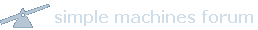September 20, 2019, 12:53:46 PM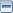News: If you like a topic and you see an orange "bump" link, click it. More info.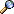Home Help Search Login Register More
 Pages:Author Topic: ECDSA math questions  (Read 503 times)
amaclin1
Sr. Member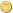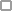Offline

Activity: 686
Merit: 271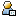November 04, 2018, 02:01:29 PM

1) is it possible to recover non-compressed key from compressed one?
for example: given compressed public key ( this one is well-known CHBS )
0378D430274F8C5EC1321338151E9F27F4C676A008BDF8638D07C0B6BE9AB35C71
need to get
0478D430274F8C5EC1321338151E9F27F4C676A008BDF8638D07C0B6BE9AB35C71A1518063243AC D4DFE96B66E3F2EC8013C8E072CD09B3834A19F81F659CC3455

2) is it possible to recover `message digest` from public key and signature (assume it is valid)?Bitcoin SV GUI client for Windows and Linux
https://github.com/AlisterMaclin/bitcoin-sv/releases
15689840261568984026

1568984026
 Report to moderator
Advertised sites are not endorsed by the Bitcoin Forum. They may be unsafe, untrustworthy, or illegal in your jurisdiction. Advertise here.
15689840261568984026

1568984026
 Report to moderator
arulbero
Legendary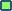Online

Activity: 1282
Merit: 1305November 04, 2018, 03:06:43 PMLast edit: November 07, 2018, 07:29:33 PM by arulberoMerited by Foxpup (5), DarkStar_ (5), suchmoon (4), aliashraf (3), LeGaulois (2), bob123 (2), Piggy (2), ETFbitcoin (1), delpiero10 (1), darosior (1)

1) is it possible to recover non-compressed key from compressed one?
for example: given compressed public key ( this one is well-known CHBS )
0378D430274F8C5EC1321338151E9F27F4C676A008BDF8638D07C0B6BE9AB35C71
need to get
0478D430274F8C5EC1321338151E9F27F4C676A008BDF8638D07C0B6BE9AB35C71A1518063243AC D4DFE96B66E3F2EC8013C8E072CD09B3834A19F81F659CC3455

Yes, it is possible.

public uncompressed key :  '04' + 'x' + 'y'

public compressed    key  : '02' or '03' + 'x'  (02 if y is even, 03 if y is odd)

curve equation y^2 = x^3 + 7  mod p
(that means that if (x,y) satisfies the equation, then (x,-y) satisfies the equation too  --> https://github.com/bitcoinbook/bitcoinbook/blob/develop/images/mbc2_0407.png )

compressed key: 0378D430274F8C5EC1321338151E9F27F4C676A008BDF8638D07C0B6BE9AB35C71
03 : y odd
78D430274F8C5EC1321338151E9F27F4C676A008BDF8638D07C0B6BE9AB35C71 : x

Then you need only to compute the y value:

(Python)
Code:
> p=0xFFFFFFFFFFFFFFFFFFFFFFFFFFFFFFFFFFFFFFFFFFFFFFFFFFFFFFFEFFFFFC2F
>
> x=0x78D430274F8C5EC1321338151E9F27F4C676A008BDF8638D07C0B6BE9AB35C71
>
> x3=pow(x,3,p)    --> x^3 = x^3 mod p
>
> y2=(x3+7) % p   --> y^2 = x^3 + 7 mod p
>
> y=pow(y2,(p+1)/4,p)  --> this line computes sqrt(y^2) = y
>
> hex(y)
'0x5eae7f9cdbc532b201694991c0d137fec371f8d32f64c7cb5e607e08a633c7da'
>
because this y is even, we compute -y = p-y (if y is even, p-y is always odd and viceversa)
>
> hex(p-y)
>'0xa1518063243acd4dfe96b66e3f2ec8013c8e072cd09b3834a19f81f659cc3455'
then: A1518063243ACD4DFE96B66E3F2EC8013C8E072CD09B3834A19F81F659CC3455 : y (odd)

uncompressed key = '04' + 'x' + 'y'

Code:
0478D430274F8C5EC1321338151E9F27F4C676A008BDF8638D07C0B6BE9AB35C71A1518063243ACD4DFE96B66E3F2EC8013C8E072CD09B3834A19F81F659CC3455

2) is it possible to recover `message digest` from public key and signature (assume it is valid)?

No, I think it is not possible.
Let (r,s) be the signature, m the message, e = H(m) the message digest, G the curve generator, d the private key, P=d*G the public key.

ECDSA works this way:
1) pick a random nonce k (a private key you use once) in [1,n-1]
2) k -> k*G = Q(xq,yq)   then r = xq mod n (r is more or less like the x coordinate of Q)
3) s = k^-1 * (r*d + e)  mod n

Then k*s = (r*d + e) mod n --> e = (s*k - r*d)  mod n     you know r and s (the signature) but not k and s (the two private keys), then you cannot retrieve the value of 'e'. (Usually you know 'e' too, in that case if you found out k, you could get d easily or viceversa, because it would be an equation with 4 known values and only 1 unknown.)

You could retrieve only e*G, because:

e*G = s*k*G - r*d*G = s*Q - r*P  mod n,  but again from e*G you can't retrieve the message digest 'e' (it would be like getting a private key from the public one).

Usually, when you verify a signature, you know 'e', and s*Q = e*G + r*P mod n -> Q = s^-1*e*G + s^-1*r*P, the last equation can be verified by knowing r, s, e, P.digitalcitizen
Copper Member
Jr. MemberOffline

Activity: 115
Merit: 4November 20, 2018, 04:10:14 AMMerited by o_e_l_e_o (1)

(Python)
Code:
> p=0xFFFFFFFFFFFFFFFFFFFFFFFFFFFFFFFFFFFFFFFFFFFFFFFFFFFFFFFEFFFFFC2F
>
> x=0x78D430274F8C5EC1321338151E9F27F4C676A008BDF8638D07C0B6BE9AB35C71
>
> x3=pow(x,3,p)    --> x^3 = x^3 mod p
>
> y2=(x3+7) % p   --> y^2 = x^3 + 7 mod p
>
> y=pow(y2,(p+1)/4,p)  --> this line computes sqrt(y^2) = y
>
> hex(y)
'0x5eae7f9cdbc532b201694991c0d137fec371f8d32f64c7cb5e607e08a633c7da'
>
because this y is even, we compute -y = p-y (if y is even, p-y is always odd and viceversa)
>
> hex(p-y)
>'0xa1518063243acd4dfe96b66e3f2ec8013c8e072cd09b3834a19f81f659cc3455'
then: A1518063243ACD4DFE96B66E3F2EC8013C8E072CD09B3834A19F81F659CC3455 : y (odd)

uncompressed key = '04' + 'x' + 'y'

Code:
0478D430274F8C5EC1321338151E9F27F4C676A008BDF8638D07C0B6BE9AB35C71A1518063243ACD4DFE96B66E3F2EC8013C8E072CD09B3834A19F81F659CC3455

Wow, thank you for posting this.  I was driving myself insane trying to understand more of the math and how it's actually implemented, trying very small values from links like this one:

https://www.coindesk.com/math-behind-bitcoin

to get a feel for it.  I think I'm getting there.  I hope that ordering and reading Mastering Bitcoin: Programming the Open Blockchain will help with the math, and trying to write my own blockchain parser.

I'm not a math heavyweight, so I have a couple of questions if you have time:

From what I understand so far, there are constants that are always the same in Bitcoin.  This includes the Q (curve generator), the p (for mod p), taken from your code and which I noted in the link to coindesk.  I couldn't see how the order was calculated, given other values.  Can you briefly describe what a base point is?  I think I'm getting a decent idea of what a finite field is.

When trying to find:

Code:
y=pow(y2,(p+1)/4,p)  --> this line computes sqrt(y^2) = y

Is this always how it's done, for any y2, p is always the same, and the (p+1)/4 part is constant as well for getting y from y2?

Thanks again!Coding Enthusiast
Hero MemberOffline

Activity: 666
Merit: 1031Novice C♯ Coder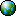November 20, 2018, 05:20:46 AMLast edit: November 20, 2018, 05:36:37 AM by Coding EnthusiastMerited by NeuroticFish (3), ETFbitcoin (1)

I hope that ordering and reading Mastering Bitcoin: Programming the Open Blockchain will help with the math, and trying to write my own blockchain parser.
FWIW you don't need to know "the math behind bitcoin" to write a blockchain parser.

This includes the Q (curve generator),
It is called "G" for Generator not Q!

I couldn't see how the order was calculated, given other values.
Order (or n) is the order of the generator or G.
Order of a point (G is a point on the curve) is the smallest positive integer such that nP = O.

Can you briefly describe what a base point is?
Base point is a point on the curve which is used in public key cryptography.

When trying to find:
Code:
y=pow(y2,(p+1)/4,p)  --> this line computes sqrt(y^2) = y
Is this always how it's done, for any y2, p is always the same, and the (p+1)/4 part is constant as well for getting y from y2?
What you need to have in mind is that calculations used in Elliptic Curve Cryptography are not regular arithmetic. Instead they are Modular Arithmetic. So y2=... is in fact y2=... mod p.
Calculating it requires using special algorithms one example is Tonelli–Shanks algorithm. There are special cases which can speed up the calculation. In case of secp256k1 elliptic curve that bitcoin is using, the prime number has a special characteristic which makes the calculation easier. And that is the fact that p%4=3 so you can use that formula above with (p+1/4 mod p)Projects List+Suggestion boxDonation link using BIP21Bech32 Donation link! BitcoinTransactionTool (0.9.2):  Ann - Source CodeWatch Only Bitcoin Wallet (supporting SegWit) (3.1.0):  Ann - Source CodeSharpPusher (broadcast transactions) (0.10.0): Ann - Source Code
digitalcitizen
Copper Member
Jr. MemberOffline

Activity: 115
Merit: 4November 20, 2018, 06:18:26 AM

FWIW you don't need to know "the math behind bitcoin" to write a blockchain parser.

Yes.  I just want to know a bit about the math for personal interest.  If that book can help a bit, then good.  Otherwise my primary interest is in writing a blockchain parser, with respect to the book.

It is called "G" for Generator not Q!

Thanks, I'm tired... haha.  But I still needed that pointed out...

I read somewhere that G is the same for everyone in Bitcoin.  I am guessing this means the Generator and its properties, not that it's a scalar or some type of simple constant.  Like you say, G is a point on a curve, and everyone uses the same curve.

Order (or n) is the order of the generator or G.
Order of a point (G is a point on the curve) is the smallest positive integer such that nP = O.

So with G point on the curve, P, n is the smallest positive integer that satisfies nP = O where O is the order.

Base point is a point on the curve which is used in public key cryptography.

This seems to be the same, but with or without a prefix of 04.  So this is a starting point to generate other points on the curve?  Oh hang on.  I'll get a book on crypto.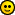What you need to have in mind is that calculations used in Elliptic Curve Cryptography are not regular arithmetic. Instead they are Modular Arithmetic. So y2=... is in fact y2=... mod p.

Calculating it requires using special algorithms one example is TonelliShanks algorithm. There are special cases which can speed up the calculation. In case of secp256k1 elliptic curve that bitcoin is using, the prime number has a special characteristic which makes the calculation easier. And that is the fact that p%4=3 so you can use that formula above with (p+1/4 mod p)

Thanks!  I appreciate the response.  That was illuminating.  I tried the Python code and it's becoming more concrete.

I'll have to go out now and dig around a little more.  But if it gets too hard, I'll stick to the coding then, and concentrate on a blockchain parser instead of trying to understand everything...Coding Enthusiast
Hero MemberOffline

Activity: 666
Merit: 1031Novice C♯ CoderNovember 20, 2018, 07:53:36 AM

I read somewhere that G is the same for everyone in Bitcoin.  I am guessing this means the Generator and its properties, not that it's a scalar or some type of simple constant.  Like you say, G is a point on a curve, and everyone uses the same curve.

In order for the elliptic curve cryptography to work we all need to use the same Elliptic Curve. And that curve is defined by a sextuple T = (p, a, b, G, n, h), so yeah G is defined by the curve and is the same when using the same curve.

So with G point on the curve, P, n is the smallest positive integer that satisfies nP = O where O is the order.

No, O is defined as the point at infinity.

This seems to be the same, but with or without a prefix of 04.  So this is a starting point to generate other points on the curve?  Oh hang on.  I'll get a book on crypto.If you want to understand why there are two public keys (with 0x04 and 0x02/0x03) read this comment:
https://bitcointalk.org/index.php?topic=5049111.msg46800542#msg46800542Projects List+Suggestion boxDonation link using BIP21Bech32 Donation link! BitcoinTransactionTool (0.9.2):  Ann - Source CodeWatch Only Bitcoin Wallet (supporting SegWit) (3.1.0):  Ann - Source CodeSharpPusher (broadcast transactions) (0.10.0): Ann - Source Code
digitalcitizen
Copper Member
Jr. MemberOffline

Activity: 115
Merit: 4November 20, 2018, 11:48:09 AM

I read somewhere that G is the same for everyone in Bitcoin.  I am guessing this means the Generator and its properties, not that it's a scalar or some type of simple constant.  Like you say, G is a point on a curve, and everyone uses the same curve.

In order for the elliptic curve cryptography to work we all need to use the same Elliptic Curve. And that curve is defined by a sextuple T = (p, a, b, G, n, h), so yeah G is defined by the curve and is the same when using the same curve.

So with G point on the curve, P, n is the smallest positive integer that satisfies nP = O where O is the order.

No, O is defined as the point at infinity.

In order for the elliptic curve cryptography to work we all need to use the same Elliptic Curve. And that curve is defined by a sextuple T = (p, a, b, G, n, h), so yeah G is defined by the curve and is the same when using the same curve.

So with G point on the curve, P, n is the smallest positive integer that satisfies nP = O where O is the order.

No, O is defined as the point at infinity.

So, we are aiming for the number of times a point can be added to itself so the slope is infinite? i.e. if you looked at a simplified graph, the slope of the line is vertical, or nearly vertical.  Or the order is some way you can detect the number of times a point addition can be done to itself before you hit O (infinity).  Is the order selected after a number of point additions, or is there an algorithm that gives you an order after repeated point doubling?  (Is that the same as adding a point to itself?)

This seems to be the same, but with or without a prefix of 04.  So this is a starting point to generate other points on the curve?  Oh hang on.  I'll get a book on crypto.If you want to understand why there are two public keys (with 0x04 and 0x02/0x03) read this comment:
https://bitcointalk.org/index.php?topic=5049111.msg46800542#msg46800542

Thanks, that was a very accessible post!Coding Enthusiast
Hero MemberOffline

Activity: 666
Merit: 1031Novice C♯ CoderNovember 20, 2018, 01:38:31 PM

My knowledge about point at infinity is a little shaky. Try reading this https://trustica.cz/en/2018/03/29/elliptic-curves-point-at-infinity/ it may help.Projects List+Suggestion boxDonation link using BIP21Bech32 Donation link! BitcoinTransactionTool (0.9.2):  Ann - Source CodeWatch Only Bitcoin Wallet (supporting SegWit) (3.1.0):  Ann - Source CodeSharpPusher (broadcast transactions) (0.10.0): Ann - Source Code
aplistir
Full MemberOffline

Activity: 351
Merit: 161November 20, 2018, 03:39:00 PMLast edit: November 20, 2018, 06:51:40 PM by aplistir

No, O is defined as the point at infinity.

So, we are aiming for the number of times a point can be added to itself so the slope is infinite? i.e. if you looked at a simplified graph, the slope of the line is vertical, or nearly vertical.  Or the order is some way you can detect the number of times a point addition can be done to itself before you hit O (infinity).  Is the order selected after a number of point additions, or is there an algorithm that gives you an order after repeated point doubling?  (Is that the same as adding a point to itself?)

No no no,
you are mixing concepts of the real word with the finite field.
In the real world there is NO infinity (in elliptic curve calculations) You can forever keep doing the additions, and you will NEVER reach infinity.

In the finite field you will reach infinity, when you have to divide by 0 (in the equations).

The order is just the number of different points in the finite field. When you do additions in the finite field, you will go through them one by one, until you have gone through all of them. the last point will be infinity, because you have to divide by zero.

In finite fields, no matter what is your generator point, the point of infinity is the last point, after that the calculations start from the beginning.

------------------
Edit:
In numerical terms, the infinity always happens when the 2 points you are trying to add together are G and -G, two points with the same x coordinate. When calculating the slope s=(y2-y1)/(x2-x1),  the x values are the same, and you cant divide by 0

In real world this cannot happen, as there is no point X in the curve that would get you to -G when adding G to X.arulbero
LegendaryOnline

Activity: 1282
Merit: 1305December 27, 2018, 04:26:31 PMLast edit: December 27, 2018, 05:05:06 PM by arulberoMerited by Piggy (1)

Let (r,s) be the signature, m the message, e = H(m) the message digest, G the curve generator, d the private key, P=d*G the public key.

ECDSA works this way:
1) pick a random nonce k (a private key you use once) in [1,n-1]
2) k -> k*G = Q(xq,yq)   then r = xq mod n (r is more or less like the x coordinate of Q)
3) s = k^-1 * (r*d + e)  mod n

Then k*s = (r*d + e) mod n --> e = (s*k - r*d)  mod n     you know r and s (the signature) but not k and s (the two private keys), then you cannot retrieve the value of 'e'. (Usually you know 'e' too, in that case if you found out k, you could get d easily or viceversa, because it would be an equation with 4 known values and only 1 unknown.)

You could retrieve only e*G, because:

e*G = s*k*G - r*d*G = s*Q - r*P  mod n,  but again from e*G you can't retrieve the message digest 'e' (it would be like getting a private key from the public one).

Usually, when you verify a signature, you know 'e', and s*Q = e*G + r*P mod n -> Q = s^-1*e*G + s^-1*r*P, the last equation can be verified by knowing r, s, e, P.

Let's give an example.

From here: http://www.righto.com/2014/02/bitcoins-hard-way-using-raw-bitcoin.html

we see how this tx was constructed

we get this data:

Code:
>>> n=0xFFFFFFFFFFFFFFFFFFFFFFFFFFFFFFFEBAAEDCE6AF48A03BBFD25E8CD0364141 (this is the group order)
>>>
>>> d=0x0caecf01d74102a28aed6a64dcf1cf7b0e41c4dd6c62f70f46febdc32514f0bd (the private key)
>>> e=0x5fda68729a6312e17e641e9a49fac2a4a6a680126610af573caab270d232f850 (the double hash of the tx/message)
>>>
>>> r=0x2cb265bf10707bf49346c3515dd3d16fc454618c58ec0a0ff448a676c54ff713 (the first part of the signature)
>>> s=0x6c6624d762a1fcef4618284ead8f08678ac05b13c84235f1654e6ad168233e82 (the second part of the signature)

Now we try to retrieve the random nonce k  (remember,  k -> k*G = Q(xq,yq)  then r = xq mod n , namely r is the x coordinate of Q, a sort of a public key, and k is its private key)

e = (s*k - r*d)  mod n

s*k = (e + r*d) mod n

k = s^-1 * (e + r*d) mod n

Code:
>>> s_inv=pow(s,n-2,n)  --> we are using the Fermat's little theorem to compute 1/s
>>> hex(s_inv)
'0x947f7b9fc41f37348c3a53a146757b9759e79da5fe039a35a9024bec1fb0ea0cL'
>>>
>>> hex((s_inv * s) % n)  --> we check that s_inv * s = 1
'0x1L'
>>>
>>> k=(s_inv * (e + r*d)) % n
>>> hex(k)
'0x4526b5ab1973ab68afd06517996d22823441dd9ef52663a57de11d6642e961e7L'

k is supposed to be the private key to r.

To have a confirm let's put  4526b5ab1973ab68afd06517996d22823441dd9ef52663a57de11d6642e961e7  here and

we get the public key

Code:
04
X = 2CB265BF10707BF49346C3515DD3D16FC454618C58EC0A0FF448A676C54FF713
Y = B317A494AB75813F8737AC779BDF68AC5235C683A0FF92AEB81655DE137FB862

As expected, X = r !Pages: